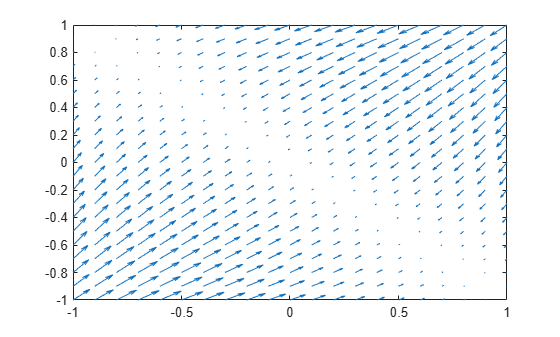Gradient vector of symbolic scalar field

## Syntax

``g = gradient(f,v)``
``g = gradient(f)``

## Description

example

````g = gradient(f,v)` returns the gradient vector of symbolic scalar field `f` with respect to vector `v` in Cartesian coordinates.```
````g = gradient(f)` returns the gradient vector of the scalar field `f` with respect to a default vector constructed from the symbolic variables in `f`.```

## Examples

collapse all

The gradient of a scalar function `f` with respect to the vector `v` is the vector of the first partial derivatives of `f` with respect to each element of `v`.

Find the gradient vector of `f(x,y,z)` with respect to vector `[x,y,z]`. The gradient is a vector with these components.

```syms x y z f(x,y,z) = 2*y*z*sin(x) + 3*x*sin(z)*cos(y); v = [x,y,z]; gradient(f,v)```
```ans(x, y, z) =  $\left(\begin{array}{c}3 \mathrm{cos}\left(y\right) \mathrm{sin}\left(z\right)+2 y z \mathrm{cos}\left(x\right)\\ 2 z \mathrm{sin}\left(x\right)-3 x \mathrm{sin}\left(y\right) \mathrm{sin}\left(z\right)\\ 2 y \mathrm{sin}\left(x\right)+3 x \mathrm{cos}\left(y\right) \mathrm{cos}\left(z\right)\end{array}\right)$```

Find the gradient of a function `f(x,y)`, and plot it as a quiver (velocity) plot.

Find the gradient vector of `f(x,y)` with respect to vector `[x,y]`. The gradient is vector `g` with these components.

```syms x y f = -(sin(x) + sin(y))^2; v = [x y]; g = gradient(f,v)```
```g =  $\left(\begin{array}{c}-2 \mathrm{cos}\left(x\right) \left(\mathrm{sin}\left(x\right)+\mathrm{sin}\left(y\right)\right)\\ -2 \mathrm{cos}\left(y\right) \left(\mathrm{sin}\left(x\right)+\mathrm{sin}\left(y\right)\right)\end{array}\right)$```

Now plot the vector field defined by these components. MATLAB® provides the `quiver` plotting function for this task. The function does not accept symbolic arguments. First, replace symbolic variables in expressions for components of `g` with numeric values. Then use `quiver`.

```[X,Y] = meshgrid(-1:.1:1,-1:.1:1); G1 = subs(g(1),v,{X,Y}); G2 = subs(g(2),v,{X,Y}); quiver(X,Y,G1,G2)```Since R2021b

Use symbolic matrix variables to define a matrix multiplication that returns a scalar.

```syms X Y [3 1] matrix A = Y.'*X```
`A = ${Y}^{\mathrm{T}} X$`

Find the gradient of the matrix multiplication with respect to $X$.

`gX = gradient(A,X)`
`gX = $Y$`

Find the gradient of the matrix multiplication with respect to $Y$.

`gY = gradient(A,Y)`
`gY = $X$`

Since R2021b

Find the gradient of the multivariable function

`$f\left(x\right)={\mathrm{sin}}^{2}\left({x}_{1,1}\right)+{\mathrm{sin}}^{2}\left({x}_{1,2}\right)+{\mathrm{sin}}^{2}\left({x}_{1,3}\right)$`

with respect to the vector $x=\left[{x}_{1,1},{x}_{1,2},{x}_{1,3}\right]$.

Use a symbolic matrix variable to express the function $f$ and its gradient in terms of the vector $x$.

```syms x [1 3] matrix f = sin(x)*sin(x).'```
`f = $\mathrm{sin}\left(x\right) {\mathrm{sin}\left(x\right)}^{\mathrm{T}}$`
`g = gradient(f,x)`
`g = $2 \left(\mathrm{cos}\left(x\right)\odot {\mathrm{I}}_{3}\right) {\mathrm{sin}\left(x\right)}^{\mathrm{T}}$`

To express the gradient in terms of the elements of $x$, convert the result to a vector of symbolic scalar variables using `symmatrix2sym`.

`g = symmatrix2sym(g)`
```g =  $\left(\begin{array}{c}2 \mathrm{cos}\left({x}_{1,1}\right) \mathrm{sin}\left({x}_{1,1}\right)\\ 2 \mathrm{cos}\left({x}_{1,2}\right) \mathrm{sin}\left({x}_{1,2}\right)\\ 2 \mathrm{cos}\left({x}_{1,3}\right) \mathrm{sin}\left({x}_{1,3}\right)\end{array}\right)$```

Alternatively, you can convert $f$ and $x$ to symbolic expressions of scalar variables and use them as inputs to the `gradient` function.

`g = gradient(symmatrix2sym(f),symmatrix2sym(x))`
```g =  $\left(\begin{array}{c}2 \mathrm{cos}\left({x}_{1,1}\right) \mathrm{sin}\left({x}_{1,1}\right)\\ 2 \mathrm{cos}\left({x}_{1,2}\right) \mathrm{sin}\left({x}_{1,2}\right)\\ 2 \mathrm{cos}\left({x}_{1,3}\right) \mathrm{sin}\left({x}_{1,3}\right)\end{array}\right)$```

Since R2023a

Create a 3-by-1 vector as a symbolic matrix variable $\mathbit{X}$. Create a scalar field that is a function of $\mathbit{X}$ as a symbolic matrix function $A\left(\mathbit{X}\right)$, keeping the existing definition of $\mathbit{X}$.

```syms X [3 1] matrix syms A(X) [1 1] matrix keepargs```

Find the gradient of $A\left(\mathbit{X}\right)$ with respect to $\mathbit{X}$. The `gradient` function returns an unevaluated formula.

`gradA = gradient(A,X)`
`gradA(X) = `

Show that the divergence of the gradient of $A\left(\mathbit{X}\right)$ is equal to the Laplacian of $A\left(\mathbit{X}\right)$, that is ${\nabla }_{\mathbit{X}}\cdot {\nabla }_{\mathbit{X}}\mathit{A}\left(\mathbit{X}\right)={\Delta }_{\mathbit{X}}\mathit{A}\left(\mathbit{X}\right)$.

`divOfGradA = divergence(gradA,X)`
`divOfGradA(X) = `
`lapA = laplacian(A,X)`
`lapA(X) = `

Show that the curl of the gradient of $A\left(\mathbit{X}\right)$ is zero, that is ${\nabla }_{\mathbit{X}}×\text{\hspace{0.17em}}{\nabla }_{\mathbit{X}}\mathit{A}\left(\mathbit{X}\right)={0}_{3,1}$.

`curlOfGradA = curl(gradA,X)`
`curlOfGradA(X) = ${\mathrm{0}}_{3,1}$`

## Input Arguments

collapse all

Symbolic scalar field, specified as a symbolic expression, symbolic function, symbolic matrix variable, or symbolic matrix function.

• If `f` is a function of symbolic scalar variables, where `f` is of type `sym` or `symfun`, then the vector `v` must be of type `sym` or `symfun`.

• If `f` is a function of symbolic matrix variables, where `f` is of type `symmatrix` or `symfunmatrix`, then the vector `v` must be of type `symmatrix` or `symfunmatrix`.

Data Types: `sym` | `symfun` | `symmatrix` | `symfunmatrix`

Vector with respect to which you find the gradient, specified as a vector of symbolic scalar variables, symbolic function, symbolic matrix variable, or symbolic matrix function.

• If you do not specify `v` and `f` is a function of symbolic scalar variables, then, by default, `gradient` constructs vector `v` from the symbolic scalar variables in `f` with the order of variables as defined by `symvar(f)`.

• If `v` is a symbolic matrix variable of type `symmatrix`, then `v` must have a size of `1`-by-`N` or `N`-by-`1`.

• If `v` is scalar, then ```gradient(f,v) = diff(f,v)```.

• If `v` is an empty symbolic object, such as `sym([])`, then `gradient` returns an empty symbolic object.

Data Types: `sym` | `symfun` | `symmatrix` | `symfunmatrix`

## Limitations

• The `gradient` function does not support tensor derivatives. If the gradient is a tensor field or a matrix rather than a vector, then the `gradient` function returns an error.

• Symbolic Math Toolbox™ currently does not support the `dot` or `cross` functions for symbolic matrix variables and functions of type `symmatrix` and `symfunmatrix`. If vector calculus identities involve dot or cross products, then the toolbox displays those identities in terms of other supported functions instead. To see a list of all the functions that support symbolic matrix variables and functions, use the commands `methods symmatrix` and `methods symfunmatrix`.

collapse all

The gradient vector of f(x) with respect to the vector $x=\left({x}_{1},{x}_{2},\dots ,{x}_{n}\right)$ is the vector of the first partial derivatives of f.
`${\nabla }_{x}f\left(x\right)=\left(\frac{\partial f}{\partial {x}_{1}},\frac{\partial f}{\partial {x}_{2}},\dots ,\frac{\partial f}{\partial {x}_{n}}\right)$`